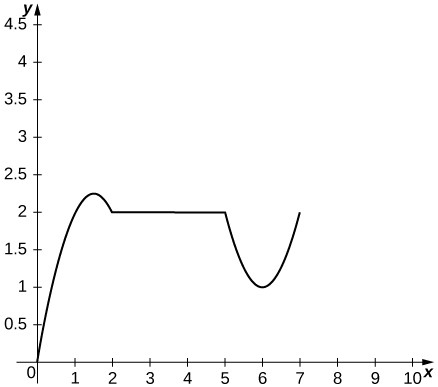$$\newcommand{\id}{\mathrm{id}}$$ $$\newcommand{\Span}{\mathrm{span}}$$ $$\newcommand{\kernel}{\mathrm{null}\,}$$ $$\newcommand{\range}{\mathrm{range}\,}$$ $$\newcommand{\RealPart}{\mathrm{Re}}$$ $$\newcommand{\ImaginaryPart}{\mathrm{Im}}$$ $$\newcommand{\Argument}{\mathrm{Arg}}$$ $$\newcommand{\norm}{\| #1 \|}$$ $$\newcommand{\inner}{\langle #1, #2 \rangle}$$ $$\newcommand{\Span}{\mathrm{span}}$$

# 2.3E: Exercises

$$\newcommand{\vecs}{\overset { \rightharpoonup} {\mathbf{#1}} }$$ $$\newcommand{\vecd}{\overset{-\!-\!\rightharpoonup}{\vphantom{a}\smash {#1}}}$$$$\newcommand{\id}{\mathrm{id}}$$ $$\newcommand{\Span}{\mathrm{span}}$$ $$\newcommand{\kernel}{\mathrm{null}\,}$$ $$\newcommand{\range}{\mathrm{range}\,}$$ $$\newcommand{\RealPart}{\mathrm{Re}}$$ $$\newcommand{\ImaginaryPart}{\mathrm{Im}}$$ $$\newcommand{\Argument}{\mathrm{Arg}}$$ $$\newcommand{\norm}{\| #1 \|}$$ $$\newcommand{\inner}{\langle #1, #2 \rangle}$$ $$\newcommand{\Span}{\mathrm{span}}$$ $$\newcommand{\id}{\mathrm{id}}$$ $$\newcommand{\Span}{\mathrm{span}}$$ $$\newcommand{\kernel}{\mathrm{null}\,}$$ $$\newcommand{\range}{\mathrm{range}\,}$$ $$\newcommand{\RealPart}{\mathrm{Re}}$$ $$\newcommand{\ImaginaryPart}{\mathrm{Im}}$$ $$\newcommand{\Argument}{\mathrm{Arg}}$$ $$\newcommand{\norm}{\| #1 \|}$$ $$\newcommand{\inner}{\langle #1, #2 \rangle}$$ $$\newcommand{\Span}{\mathrm{span}}$$

## Exercise $$\PageIndex{1}$$

For the following exercises, the given functions represent the position of a particle traveling along a horizontal line.

a. Find the velocity and acceleration functions.

b. Determine the time intervals when the object is slowing down or speeding up.

1) $$s(t)=2t^3−3t^2−12t+8$$

2) $$s(t)=2t^3−15t^2+36t−10$$

1a. $$v(t)=6t^2−30t+36,a(t)=12t−30$$

b. speeds up $$(2,2.5)∪(3,∞)$$, slows down $$(0,2)∪(2.5,3)$$

## Exercise $$\PageIndex{2}$$

A rocket is fired vertically upward from the ground. The distance s in feet that the rocket travels from the ground after t seconds is given by $$s(t)=−16t^2+560t$$.

a. Find the velocity of the rocket 3 seconds after being fired.

b. Find the acceleration of the rocket 3 seconds after being fired.

a. $$464ft/s^2$$

b.$$−32ft/s^2$$

## Exercise $$\PageIndex{3}$$

A ball is thrown downward with a speed of 8 ft/s from the top of a 64-foot-tall building. After t seconds, its height above the ground is given by $$s(t)=−16t^2−8t+64.$$

a. Determine how long it takes for the ball to hit the ground.

b. Determine the velocity of the ball when it hits the ground.

Under Construction

## Exercise $$\PageIndex{4}$$

The position function $$s(t)=t^2−3t−4$$ represents the position of the back of a car backing out of a driveway and then driving in a straight line, where s is in feet and t is in seconds. In this case, $$s(t)=0$$ represents the time at which the back of the car is at the garage door, so $$s(0)=−4$$ is the starting position of the car, 4 feet inside the garage.

a. Determine the velocity of the car when $$s(t)=0$$.

b. Determine the velocity of the car when $$s(t)=14$$.

a. $$5 ft/s$$

b. $$9 ft/s$$

## Exercise $$\PageIndex{5}$$

7) The position of a hummingbird flying along a straight line in t seconds is given by $$s(t)=3t^3−7t$$ meters.

a. Determine the velocity of the bird at $$t=1$$ sec.

b. Determine the acceleration of the bird at $$t=1$$ sec.

c. Determine the acceleration of the bird when the velocity equals 0.

Under Construction

## Exercise $$\PageIndex{6}$$

A potato is launched vertically upward with an initial velocity of 100 ft/s from a potato gun at the top of an 85-foot-tall building. The distance in feet that the potato travels from the ground after $$t$$ seconds is given by $$s(t)=−16t^2+100t+85$$.

a. Find the velocity of the potato after $$0.5s$$ and $$5.75s$$.

b. Find the speed of the potato at 0.5 s and 5.75 s.

c. Determine when the potato reaches its maximum height.

d. Find the acceleration of the potato at 0.5 s and 1.5 s.

e. Determine how long the potato is in the air.

f. Determine the velocity of the potato upon hitting the ground.

a. 84 ft/s, −84 ft/s

b. 84 ft/s

c. $$\frac{25}{8}s$$

d. $$−32ft/s^2$$ in both cases

e. $$\frac{1}{8}(25+\sqrt{965})s$$

f. $$−4\sqrt{965}ft/s$$

## Exercise $$\PageIndex{7}$$

The position function $$s(t)=t^3−8t$$ gives the position in miles of a freight train where east is the positive direction and $$t$$ is measured in hours.

a. Determine the direction the train is traveling when $$s(t)=0$$.

b. Determine the direction the train is traveling when $$a(t)=0$$.

c. Determine the time intervals when the train is slowing down or speeding up.

Under Construction

## Exercise $$\PageIndex{8}$$

The following graph shows the position $$y=s(t)$$ of an object moving along a straight line.a. Use the graph of the position function to determine the time intervals when the velocity is positive, negative, or zero.

b. Sketch the graph of the velocity function.

c. Use the graph of the velocity function to determine the time intervals when the acceleration is positive, negative, or zero.

d. Determine the time intervals when the object is speeding up or slowing down.

a. Velocity is positive on $$(0,1.5)∪(6,7)$$, negative on $$(1.5,2)∪(5,6)$$, and zero on $$(2,5)$$